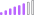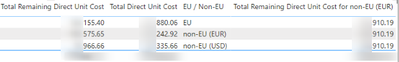cancel
Showing results for
Did you mean:

Fabric is Generally Available. Browse Fabric Presentations. Work towards your Fabric certification with the Cloud Skills Challenge.Post Patron

## IF statement won't work properly

I have written a long if statement that goes as followed:

``````Total Direct Unit Cost =
VAR CurrencyUSD = CALCULATE(SUM('Currency Rate'[Units per EUR]),'Currency Rate'[Currency] = "USD")
RETURN

IF(CALCULATE(SUM('Stock Forecast'[Total Remaining Direct Unit Cost]),
'Vendor List'[EU / Non-EU] IN { "EU" }), SUM('Stock Forecast'[Total Remaining Direct Unit Cost])*1.03,

IF (CALCULATE(SUM('Stock Forecast'[Total Remaining Direct Unit Cost]), 'Vendor List'[EU / Non-EU] IN { "non-EU (EUR)" }), SUM('Stock Forecast'[Total Remaining Direct Unit Cost])*1.06,

IF(CALCULATE(SUM('Stock Forecast'[Total Remaining Direct Unit Cost]),
'Vendor List'[EU / Non-EU] IN { "non-EU (USD)" }), DIVIDE(SUM('Stock Forecast'[Total Remaining Direct Unit Cost])*1.35,CurrencyUSD),

IF( ISBLANK(SUM('Stock Forecast'[Total Remaining Direct Unit Cost])) = TRUE, 0 ))))``````

Basically:

Set a var for currency rate of USD

IF 'Vendor List'[EU / Non-EU] contains "EU" then I have to take the sum of [Total Remaining Direct Unit Cost]*1.03

ELSE IF 'Vendor List'[EU/Non-EU] contains "non-EU (EUR)" then I have to take the sum of [Total Remaining Direct Unit Cost]*1.06

ELSE 'Vendor List'[EU/Non-EU] contains "non-EU (USD)" then I have to take the sum of [Total Remaining Direct Unit Cost]*1.35 / Currency Rate

Unfortunately with the code I wrote, I won't get the desired result:1: The first column is the amount without the measure.

2: The second column is the amount with the calculation written above (but calculation is wrong)

3: The third column indicates if the amount is for EU, non-EU (EUR) or non-EU (USD),

4: The fourth column is a measure with the desired result for the column that contains non-EU (EUR)

This is the measure of the fourth column:

``````CALCULATE(
SUM('Stock Forecast'[Total Remaining Direct Unit Cost]),
'Vendor List'[EU / Non-EU] IN { "non-EU (EUR)" }
)*1.06``````

How can I make sure that I get the result of the fourth column for non-EU (EUR)?

1 ACCEPTED SOLUTIONCommunity Support

Hi @Niels_T ,

Based on my understanding, try to modify your measure like so:

``````Total Direct Unit Cost =
VAR CurrencyUSD =
CALCULATE (
SUM ( 'Currency Rate'[Units per EUR] ),
'Currency Rate'[Currency] = "USD"
)
RETURN
IF (
'Vendor List'[EU / Non-EU] IN { "EU" },
SUM ( 'Stock Forecast'[Total Remaining Direct Unit Cost] ) * 1.03,
IF (
'Vendor List'[EU / Non-EU] IN { "non-EU (EUR)" },
SUM ( 'Stock Forecast'[Total Remaining Direct Unit Cost] ) * 1.06,
IF (
'Vendor List'[EU / Non-EU] IN { "non-EU (USD)" },
DIVIDE (
SUM ( 'Stock Forecast'[Total Remaining Direct Unit Cost] ) * 1.35,
CurrencyUSD
),
IF ( ISBLANK ( SUM ( 'Stock Forecast'[Total Remaining Direct Unit Cost] ) ), 0 )
)
)
)
``````

Or like so:

``````Total Direct Unit Cost =
VAR CurrencyUSD =
CALCULATE (
SUM ( 'Currency Rate'[Units per EUR] ),
'Currency Rate'[Currency] = "USD"
)
RETURN
SWITCH (
TRUE (),
SWITCH (
'Vendor List'[EU / Non-EU],
"EU", SUM ( 'Stock Forecast'[Total Remaining Direct Unit Cost] ) * 1.03,
"non-EU (EUR)", SUM ( 'Stock Forecast'[Total Remaining Direct Unit Cost] ) * 1.06,
"non-EU (USD)",
DIVIDE (
SUM ( 'Stock Forecast'[Total Remaining Direct Unit Cost] ) * 1.35,
CurrencyUSD
)
), ISBLANK ( SUM ( 'Stock Forecast'[Total Remaining Direct Unit Cost] ) ),
0
)
``````

Best Regards,

Icey

If this post helps, then please consider Accept it as the solution to help the other members find it more quickly.

2 REPLIES 2Community Support

Hi @Niels_T ,

Based on my understanding, try to modify your measure like so:

``````Total Direct Unit Cost =
VAR CurrencyUSD =
CALCULATE (
SUM ( 'Currency Rate'[Units per EUR] ),
'Currency Rate'[Currency] = "USD"
)
RETURN
IF (
'Vendor List'[EU / Non-EU] IN { "EU" },
SUM ( 'Stock Forecast'[Total Remaining Direct Unit Cost] ) * 1.03,
IF (
'Vendor List'[EU / Non-EU] IN { "non-EU (EUR)" },
SUM ( 'Stock Forecast'[Total Remaining Direct Unit Cost] ) * 1.06,
IF (
'Vendor List'[EU / Non-EU] IN { "non-EU (USD)" },
DIVIDE (
SUM ( 'Stock Forecast'[Total Remaining Direct Unit Cost] ) * 1.35,
CurrencyUSD
),
IF ( ISBLANK ( SUM ( 'Stock Forecast'[Total Remaining Direct Unit Cost] ) ), 0 )
)
)
)
``````

Or like so:

``````Total Direct Unit Cost =
VAR CurrencyUSD =
CALCULATE (
SUM ( 'Currency Rate'[Units per EUR] ),
'Currency Rate'[Currency] = "USD"
)
RETURN
SWITCH (
TRUE (),
SWITCH (
'Vendor List'[EU / Non-EU],
"EU", SUM ( 'Stock Forecast'[Total Remaining Direct Unit Cost] ) * 1.03,
"non-EU (EUR)", SUM ( 'Stock Forecast'[Total Remaining Direct Unit Cost] ) * 1.06,
"non-EU (USD)",
DIVIDE (
SUM ( 'Stock Forecast'[Total Remaining Direct Unit Cost] ) * 1.35,
CurrencyUSD
)
), ISBLANK ( SUM ( 'Stock Forecast'[Total Remaining Direct Unit Cost] ) ),
0
)
``````

Best Regards,

Icey

If this post helps, then please consider Accept it as the solution to help the other members find it more quickly.Super User

Hi @Niels_T ,

The main issue of your formula is that the IF statement is not comparing anything so the calculation is always true and returns incorrect totals.

If the information is sensitive please share it trough private message.

Regards

Miguel Félix

Proud to be a Super User!

Check out my blog: Power BI em PortuguêsAnnouncements#### Power BI Monthly Update - November 2023

Check out the November 2023 Power BI update to learn about new features.#### The largest Power BI and Fabric virtual conference

130+ sessions, 130+ speakers, Product managers, MVPs, and experts. All about Power BI and Fabric. Attend online or watch the recordings.Top Solution Authors
Top Kudoed Authors
Users online (6,631)